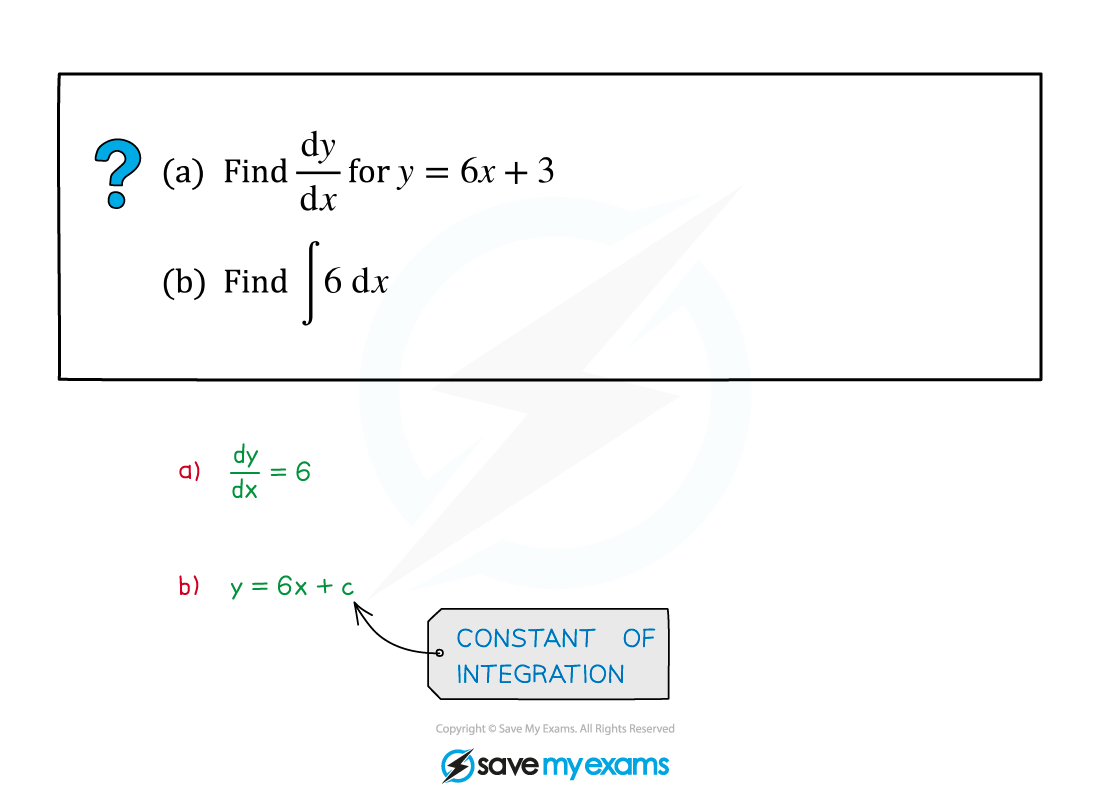# AQA A Level Maths: Pure复习笔记8.1.1 Fundamental Theorem of Calculus

### Fundamental Theorem of Calculus

#### What is the fundamental theorem of calculus?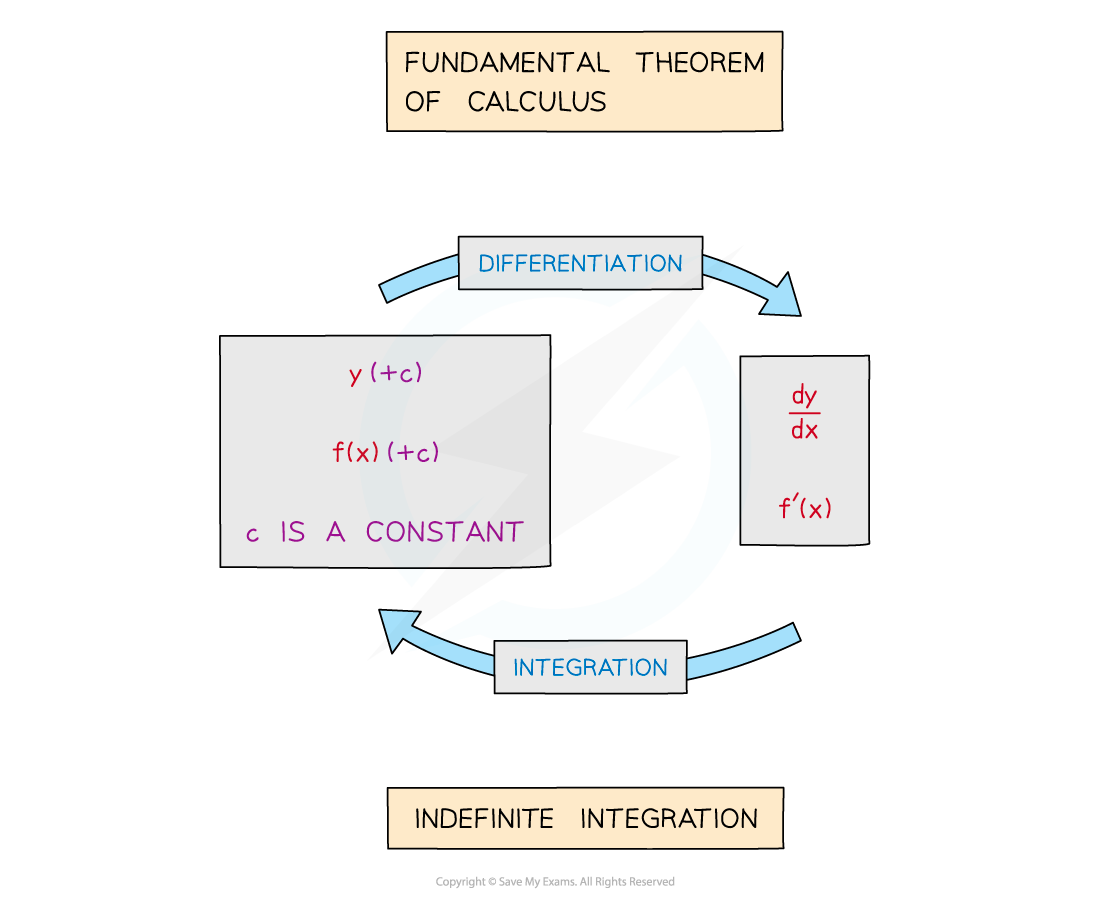• The Fundamental Theorem of Calculus states that integration is the inverse process of differentiation
• This form of the Theorem relates to Indefinite Integration
• An alternative version of the Fundamental Theorem of Calculus involves Definite Integration

#### What is “+c” (plus c)?

•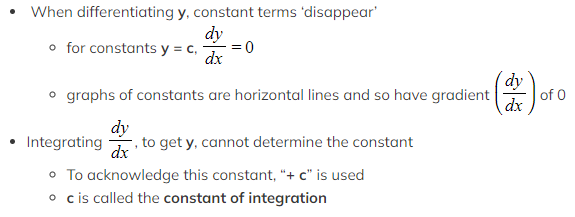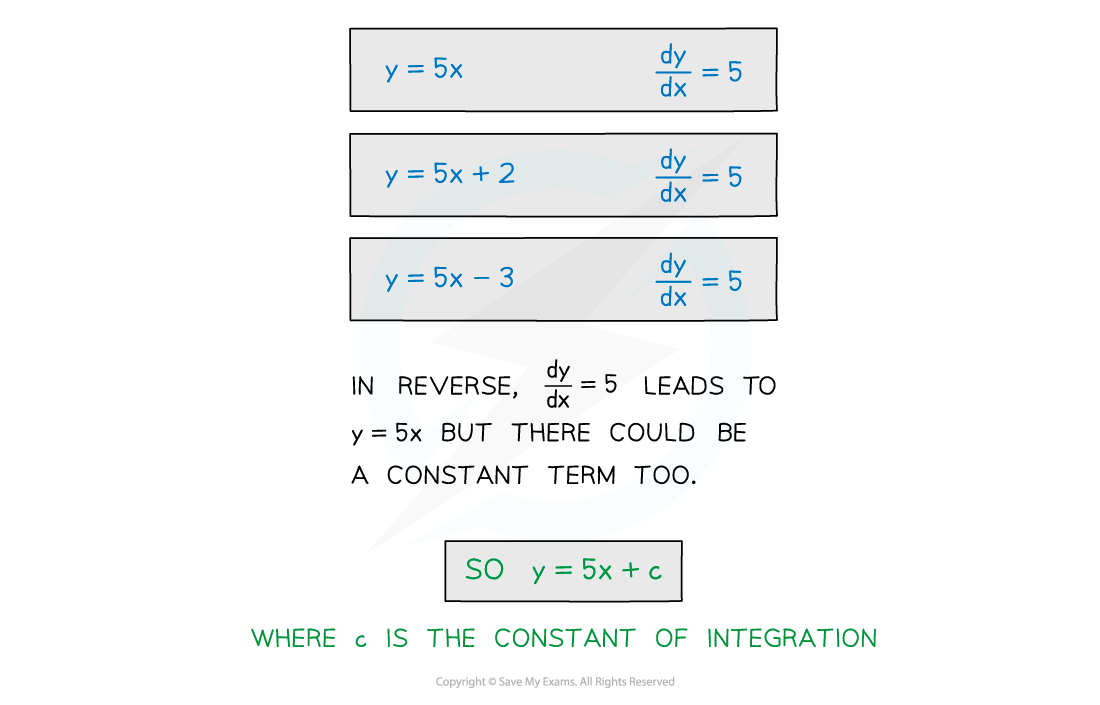#### Notation•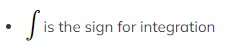If it has more than one term the function to be integrated (called the integrand) should be in brackets
• “Integrate” -–  “all of (…)”  -–  “with respect to x”
• dx means integrate with respect to x, any other letter is treated like a number (ie like a constant)

#### Worked Example